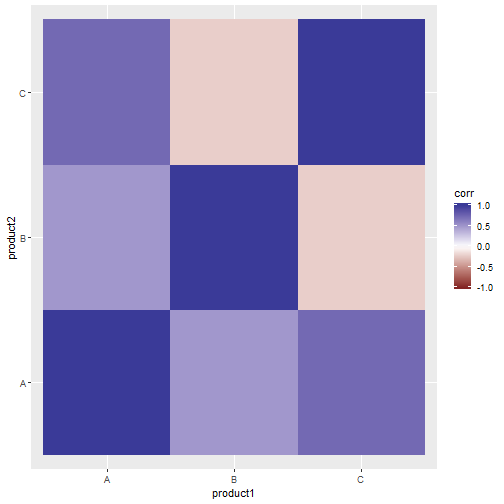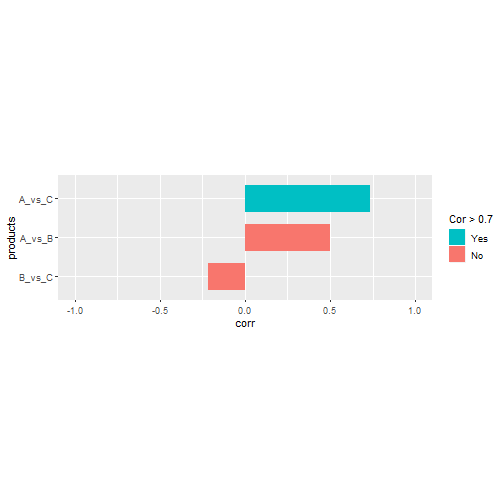# Compute correlations using the tidyverse

This small example aims to provide some use cases for the `tidyr` package. Let’s generate some example data first:

We want to compute the correlation of the sales from products A, B and C. The base R function `cor()` takes a matrix or data.frame and computes the correlation between all the column pairs. Thus, first we need to convert the data.frame `sales`, which is in long form, to wide form with one column per product.

To manipulate the correlation matrix using `tidyverse`-related functions we need to convert back the previous matrix to a long data.frame:

Now we can plot the correlation matrix using ggplot2, for instance with a heatmap:Another common way of representing correlation is a vertical barplot. For this type of plot we often want to ignore the diagonal and upper/lower triangle, and sort from lowest to highest:Here we are using a neat trick to ignore rows with duplicate product IDs ignoring its order (see this and this). The previous trick can be generalized to more than two columns, although it is not trivial (see this question for a base R solution). Let’s create first some example data:

We would like to obtain unique ID combinations without taking order into account, that is, “AAB” and “ABA” are both the same:

Note the `c(...)`, since the `.f` argument in `pmap()` is a function with as many arguments as columns in the data frame (in contrast to base `apply()`). Thus we need to collect them all in a vector, which is then sorted and finally converted into a single value with `paste(..., collapse="_")`.

Updated: